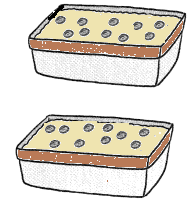# Math in Focus Grade 1 Chapter 18 Answer Key Multiplication and Division

Go through the Math in Focus Grade 1 Workbook Answer Key Chapter 18 Multiplication and Division to finish your assignments.

## Math in Focus Grade 1 Chapter 18 Answer Key Multiplication and Division

Challenging Practice

Lena collects erasers. Look at the picture. Then fill in the blanks.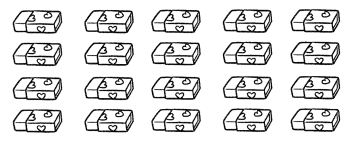Question 1.
Lena has __________ erasers.
20 erasers
Explanation:
Lena has 20 erasers.
Count the number of groups.
Then arrange the erasers in each group equally
5 fours = 20

Question 2.
She puts the erasers equally into 5 boxes. How many erasers are in each box? __________
4 erasers are in each box.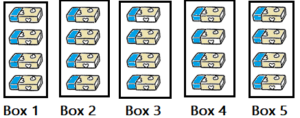Explanation:
Lena has 20 erasers.
She want to put the erasers in 5 boxes
20 ÷ 5 = 4

Question 3.
She puts the erasers equally into 4 boxes. How many erasers are in each box? __________
5 erasers are in each box.
Explanation:
Lena has 20 erasers,
she want to put in 4 boxes.
4 fives = 20
4 x 5 = 20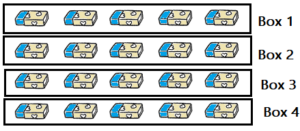Question 4.
She puts 10 erasers into each box. How many boxes does she need? __________
2 boxes does she need
Explanation:
Lena has 20 erasers,
She puts 10 erasers into each box.
10 x 2 = 20
So, she need 2 boxes.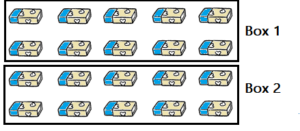Question 5.
She puts 4 erasers into each box. How many boxes does she need? __________
5 boxes she needed.
Explanation:
Lena has 20 erasers.
She puts 4 erasers into each box.
5 fours = 20
5 x 4 = 20Problem Solving

Solve.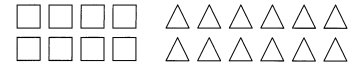There are 8 squares and 12 triangles. Put an equal number of each shape into each group.

Question 1.
Put them into 2 groups.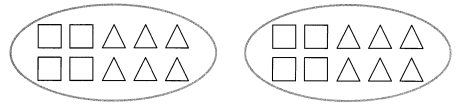There are __________ ☐ in each group.
There are __________ △ in each group.
There are __________ shapes in each group.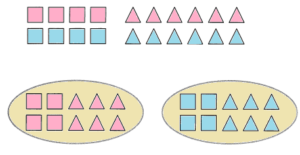There are 4 ☐ in each group.
There are 6 △ in each group.
There are (△ and ☐) 2 shapes in each group.
Explanation:
Count the number of triangles and squares.
Then put them into 2 groups equally.
Triangles = 8 ÷ 2 = 4
Squares = 8 ÷ 2 = 4
So, There are (△ and ☐) 2 shapes in each group.

Question 2.
Put them into 4 groups.
There are __________ ☐ in each group.
There are __________ △ in each group.
There are __________ shapes in each group.
There are 2 ☐ in each group.
There are 3 △ in each group.
There are (△ and ☐) 2 shapes in each group.
Explanation:
Count the number of triangles and squares.
Then put them into 4 groups equally.
Triangles = 8 ÷ 4 = 2
Squares = 8 ÷ 4 = 2
So, There are (△ and ☐) 2 shapes in each group.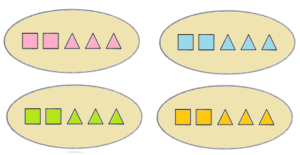Chapter Review/Test

Vocabulary

Choose the correct word.

same
groups

Question 1.The picture shows equal ____________
The picture shows equal groups
Explanation:
The above picture shows the groups,
because total number of stars are equally divided into 3 groups.
So, stars are arranged in groups.

Question 2.
By adding the ____________ number, you will have 9 ★ in all.
By adding the same number, you will have 9 ★ in all.
Explanation:
The above picture shows the groups of tar,
which are equally divided into 3 groups.
Then add the same number of stars  to the other to get the sum.

Concepts and Skills

Fill in the blanks.

Question 3.
2 + 2 + 2 = ____________
3 twos = ____________
2 + 2 + 2 = 6
3 twos = 6
Explanation:
The sum is 6,
6 is equally divided into 3 groups,
each group has 2 numbers.

Question 4.
5 + 5 + 5 + 5 = ____________
4 fives = ____________
5 + 5 + 5 + 5 = 20
4 fives =20
Explanation:
The sum is 20,
20 is equally divided into 3 groups,
each group has 5 numbers.

Match.

Question 5.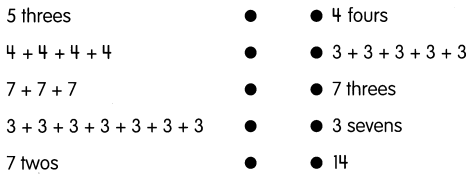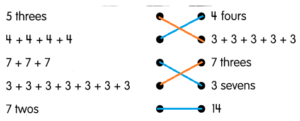Explanation:
5 threes  is written as 3+3+3+3+3
3 is arranged in 5 groups.
4+4+4+4 is written as 16
16 is divided in 4 groups.
7+7+7 is written as 7 threes,
7 is arranged in 3 groups.
3+3+3+3+3+3+3 is written as 3 sevens,
3 is arranged in 7 groups.
7 twos is written as 7+7,
7 is arranged in 2 groups.

Question 6.
A farmer has 16 chickens. He puts 8 chickens in a coop. How many coops does he need?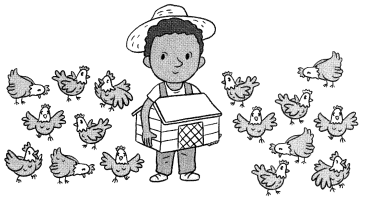He needs ____________ coops.
2 coops
Explanation:
A farmer has 16 chickens.
He puts 8 chickens in a coop.
Total coops does he need
2 coops does he need
2 eights = 16
2 x 8 = 16

Question 7.
There are 20 seeds. Put an equal number of seeds in each pot.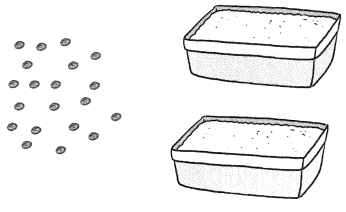There are ____________ seeds in each pot.